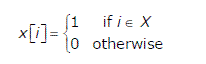# Algorithms | Misc | Question 13

• Last Updated : 28 Jun, 2021

A set X can be represented by an array x[n] as follows:Consider the following algorithm in which x,y and z are Boolean arrays of size n:

 `algorithm zzz(x[] , y[], z [])``{``   ``int` `i;``   ``for` `(i=O; i

The set Z computed by the algorithm is:
(A) (X Intersection Y)
(B) (X Union Y)
(C) (X-Y) Intersection (Y-X)
(D) (X-Y) Union (Y-X)